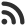Feed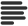Articles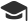Tutorials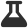CodingLab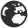CommunityLeaderboardFounder & Programmer at HackersFriend Updated Jan. 3, 2020, 8 p.m. ⋅ 68 views

# Program to find the mid-point of a line

Finding the mid point of line is easy with mid-point algorithm.

In this article, we will implement mid point algorithm to find the mid point of a line segment.

Let's suppose we are given the x and y co-ordinates of start and end point a line. Call them, x1,y1 and x2 and y2.

According to mid-point algorithm, mid-point of line is given by ((x1+ x2) / 2 , (y1+y2) / 2) , here both these will give x and y co-ordinates of mid-point resepctively.Let's code this approach:

``````// C++ program to find midpoint of a line
#include<bits/stdc++.h>
using namespace std;

void midpoint(int x1, int x2, int y1, int y2)
{
cout << (float)(x1+x2)/2 <<" , "<< (float)(y1+y2)/2 ;
}

int main()
{
int x1 =-5, y1 = 2 ;
int x2 = 3, y2 = -6 ;
midpoint(x1, x2, y1, y2);
return 0;
}
``````

``-1 , -2``

 arrow_upward UpvoteComment arrow_downward downvote

Events

Nov. 28, 2018, 5:30 p.m.

Python from zero to hero

place Delhi

Aug. 13, 2018, 5:30 p.m.

Python from zero to hero

place Bangalore ( HackersFriend office BTM Layout)